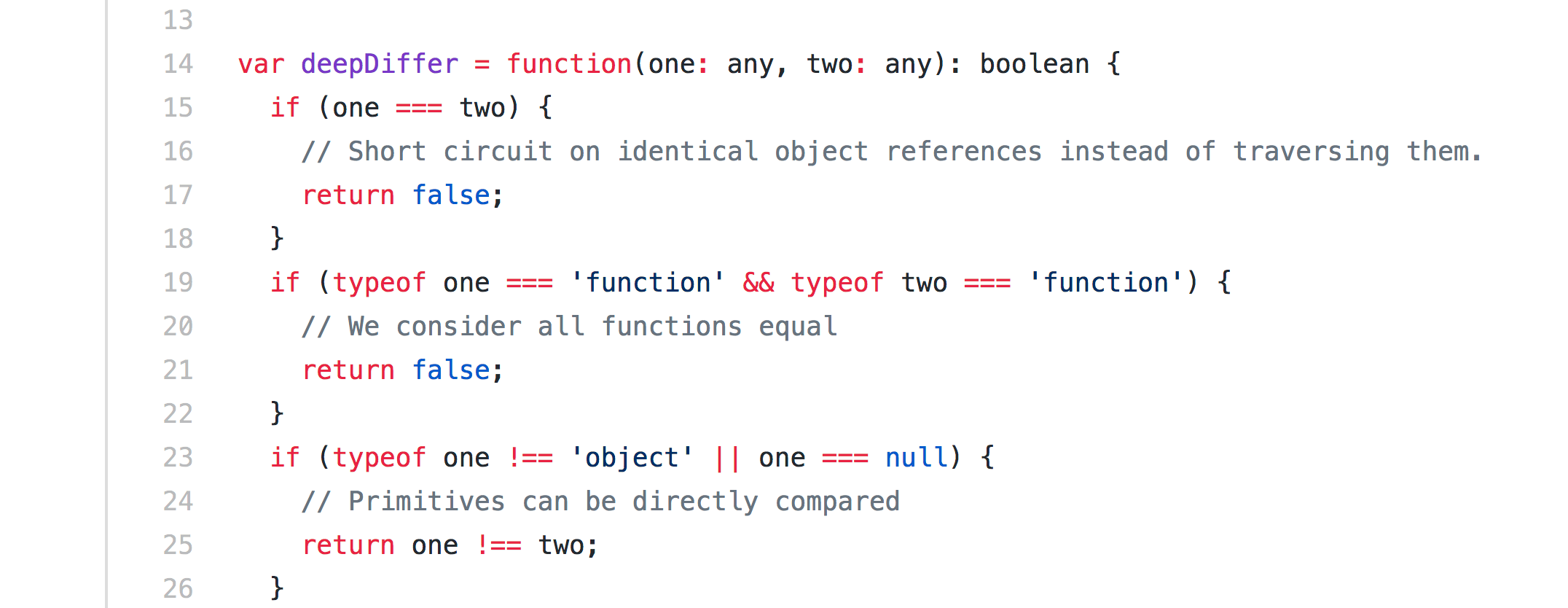# Coding Tip: Try to Code Without If-statements

## You might uncover better solutionsCode from a Facebook project. Please don’t sue them.
Update: This article is now part of my book “Learn Coding with Modern JavaScript”.
Read the updated version of it at jscomplete.com/no-ifs.

When I teach beginners to program and present them with code challenges, one of my favorite follow-up challenges is: Now solve the same problem without using if-statements (or ternary operators, or switch statements).

You might ask why would that be helpful?

Well, I think this challenge forces your brain to think differently and in some cases, the different solution might be better.

There is nothing wrong with using if-statements, but avoiding them can sometimes make the code a bit more readable to humans. This is definitely not a general rule as sometimes avoiding if-statements will make the code a lot less readable. You be the judge.

Avoiding if-statements is not just about readability. There is some science behind the concept. As the challenges below will show, not using if-statements gets you closer to the code-as-data concept, which opens the door for unique capabilities like modifying the code as it is being executed!

In all cases, it is always fun to try and solve a coding challenge without the use of any conditionals.

Here are some example challenges with their if-based solutions and if-less solutions. All solutions are written in JavaScript.

Tell me which solutions do you think are more readable.

#### Challenge #1: Count the odd integers in an `array`

Let’s say we have an array of integers and we want to count how many of these integers are odd.

Here is an example to test with:

`const arrayOfIntegers = [1, 4, 5, 9, 0, -1, 5];`

Here is a solution using an if-statement:

`let counter = 0;`
`arrayOfIntegers.forEach((integer) => {  const remainder = Math.abs(integer % 2);`
`  if (remainder === 1) {    counter++;  }});`
`console.log(counter);`

Here is a solution without if-statements:

`let counter = 0;`
`arrayOfIntegers.forEach((integer) => {  const remainder = Math.abs(integer % 2);  counter += remainder;});`
`console.log(counter);`

Note: the examples above use `forEach` and mutate the counter variable. It is cleaner and safer to use immutable methods instead. However, this article is not about that topic. Stay tuned for more coding tips articles that will cover immutability and functional programming. Also note, as pointed out by David Nagli, that the if-less solution would only work for integers while the if-based solution has the advantage of handling any number including decimal ones.

In the if-less solution, we are taking advantage of the fact that the result of modulus `2` operation is always either `1` (for odd) or `0`(for even). This result is data. We just used that data directly.

What about counting even integers? Can you think of a way to do that without using an if-statement?

#### Challenge #2: The weekendOrWeekday function

Write a function that takes a date object argument (like `new Date()`) and returns the string “weekend” or “weekday”.

Here is a solution using an if-statement:

`const weekendOrWeekday = (inputDate) => {  const day = inputDate.getDay();`
`  if (day === 0 || day === 6) {    return 'weekend';  }     return 'weekday';`
`  // Or, for ternary fans:  // return (day === 0 || day === 6) ? 'weekend' : 'weekday';};`
`console.log(weekendOrWeekday(new Date()));`

Here is a solution without if-statements:

`const weekendOrWeekday = (inputDate) => {  const day = inputDate.getDay();`
`  return weekendOrWeekday.labels[day] ||          weekendOrWeekday.labels['default'];};`
`weekendOrWeekday.labels = {   0: 'weekend',   6: 'weekend',   default: 'weekday' };`
`console.log(weekendOrWeekday(new Date()));`

Did you notice that the if-statement condition has some data in it? It tells us which days are weekends. What we did to avoid the if-statement is extract that data into an object and use that object directly. That also enables us to store that data on higher generic levels.

Update: Harald Niesche pointed out correctly that the `||` trick above is technically a conditional thing. I’ve opted to use it so that I don’t repeat “weekday” five times. We can easily get rid of it if we put the whole 7 days in the labels object.

#### Challenge #3: The doubler function (here be dragons)

Write the doubler function which, based on the type of its input, would do the following:

• When the input is a number, it doubles it (i.e. `5 => 10`, `-10 => -20`).
• When the input is a string, it repeats every letter (i.e. `'hello' => 'hheelloo'`).
• When the input is a function, it calls it twice.
• When the input is an array, it calls itself on all elements of that array.
• When the input is an object, it calls itself on all the values of that object.

Here is a solution using a switch-statement:

`const doubler = (input) => {  switch (typeof input) {    case 'number':      return input + input;    case 'string':      return input        .split('')        .map((letter) => letter + letter)        .join('');    case 'object':      Object.keys(input)            .map((key) => (input[key] = doubler(input[key])));      return input;    case 'function':      input();      input();  }};`
`console.log(doubler(-10));console.log(doubler('hey'));console.log(doubler([5, 'hello']));console.log(doubler({ a: 5, b: 'hello' }));console.log(  doubler(function() {    console.log('call-me');  }),);`

Here is a solution without a switch-statement:

`const doubler = (input) => {  return doubler.operationsByType[typeof input](input);};`
`doubler.operationsByType = {  number: (input) => input + input,  string: (input) =>    input      .split('')      .map((letter) => letter + letter)      .join(''),  function: (input) => {    input();    input();  },  object: (input) => {    Object.keys(input)          .map((key) => (input[key] = doubler(input[key])));    return input;  },};`
`console.log(doubler(-10));console.log(doubler('hey'));console.log(doubler([5, 'hello']));console.log(doubler({ a: 5, b: 'hello' }));console.log(  doubler(function() {    console.log('call-me');  }),);`

Again, notice how the data (which operations should be done for which input type) was all extracted out of the switch statement into an object. Then the object was used to pick the right operation and invoke it with the original input.

Now that you have seen a few examples, what are your thoughts? Do you have any arguments in favor or against either of these two coding styles?

Thanks for reading.